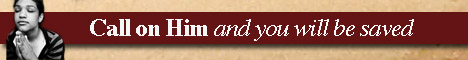Note:  Do not rely on this information. It is very old.

# Boundary

Boundary, in Geometry, means the geometrical entity that separates any other geometrical entity from its surroundings. The four geometrical entities usually dealt with are solids, surfaces, lines, and points. A solid is bounded by surfaces, a surface by lines, and a line by points. If the surfaces bounding a solid be given, the solid is completely determined. But given the close curve bounding a surface, we may obtain an infinite number of other surfaces with the same boundary. So also an infinite number of different lines may be bounded by the same two points. [Geometry.]